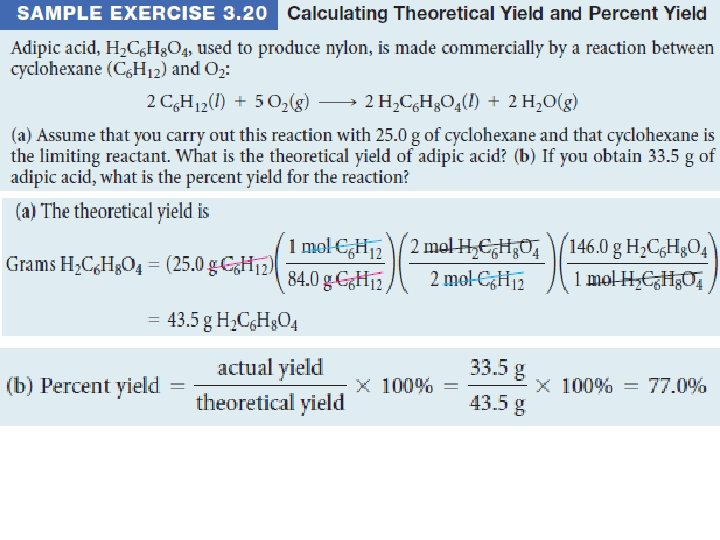Chapter 3 Stoichiometry Calculations with Chemical Formulas and

• Slides: 46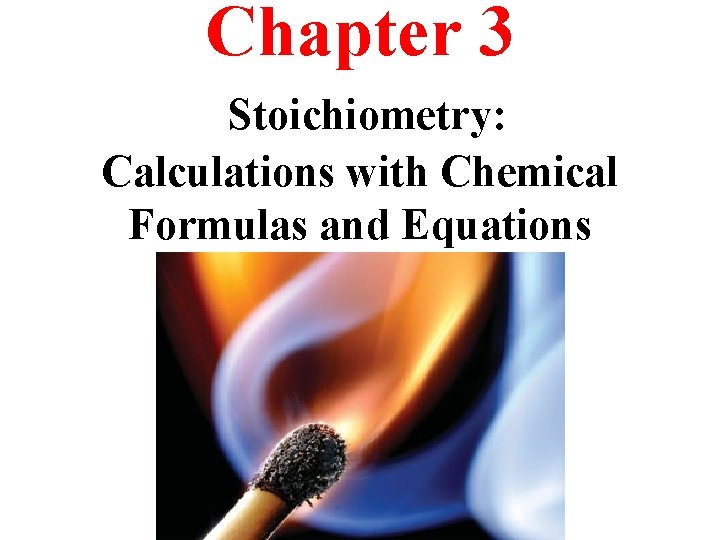Chapter 3 Stoichiometry: Calculations with Chemical Formulas and Equations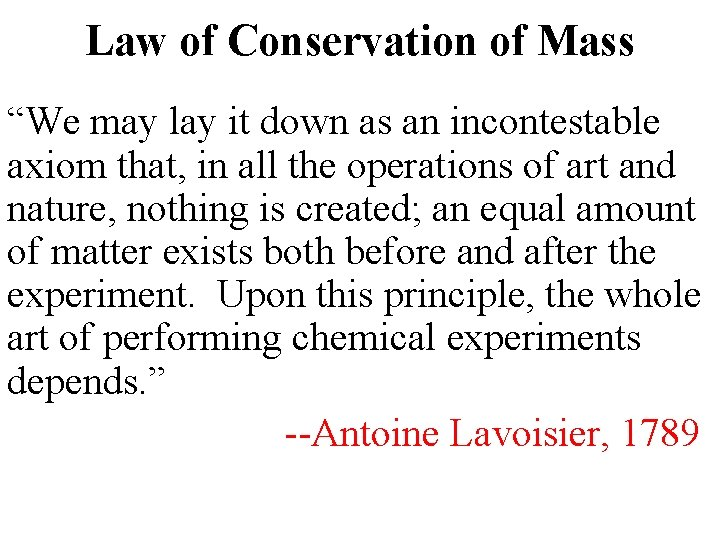Law of Conservation of Mass “We may lay it down as an incontestable axiom that, in all the operations of art and nature, nothing is created; an equal amount of matter exists both before and after the experiment. Upon this principle, the whole art of performing chemical experiments depends. ” --Antoine Lavoisier, 1789Chemical Equations Chemical equations are concise representations of chemical reactions.Anatomy of a Chemical Equation CH 4(g) + 2 O 2(g) CO 2(g) + 2 H 2 O(g) Reactants appear on the left side of the equation. Products appear on the right side of the equation. Coefficients are inserted to balance the equation.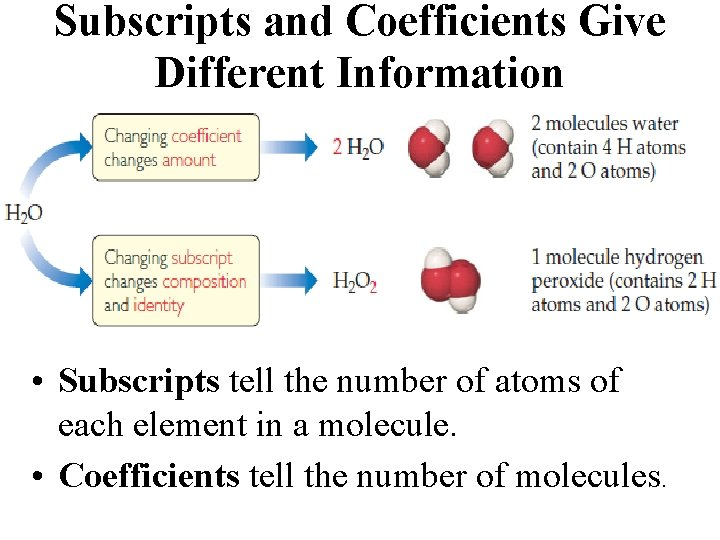Subscripts and Coefficients Give Different Information • Subscripts tell the number of atoms of each element in a molecule. • Coefficients tell the number of molecules.Reaction TypesCombination Reactions In combination reactions two or more substances react to form one product. 2 Mg(s) + O 2(g) 2 Mg. O(s) N 2(g) + 3 H 2(g) 2 NH 3(g) C 3 H 6(g) + Br 2(l) C 3 H 6 Br 2(l)Decomposition Reactions In a decomposition reaction one substance breaks down into two or more substances. Decomposition of sodium azide, Na. N 3(s), is used to inflate automobile air bags. Ca. CO 3(s) Ca. O(s) + CO 2(g) 2 KCl. O 3(s) 2 KCl(s) + O 2(g) 2 Na. N 3(s) 2 Na(s) + 3 N 2(g)Sample Exercise 3. 3 Writing Balanced Equations for Combination and Decomposition Reactions Write a balanced equation for (a) the combination reaction between lithium metal and fluorine gas and (b) the decomposition reaction that occurs when solid barium carbonate is heated (two products form, a solid and a gas). Solution: (a) 2 Li(s) + F 2(g) (b) Ba. CO 3(s) 2 Li. F(s) Ba. O(s) + CO 2(g)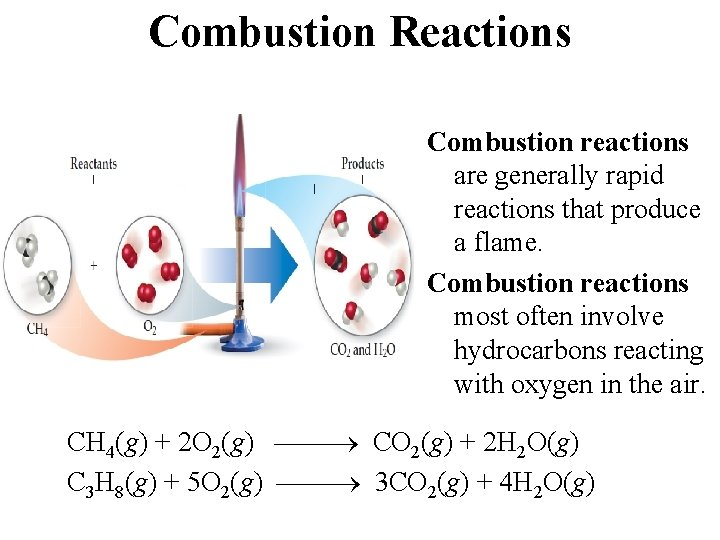Combustion Reactions Combustion reactions are generally rapid reactions that produce a flame. Combustion reactions most often involve hydrocarbons reacting with oxygen in the air. CH 4(g) + 2 O 2(g) CO 2(g) + 2 H 2 O(g) C 3 H 8(g) + 5 O 2(g) 3 CO 2(g) + 4 H 2 O(g)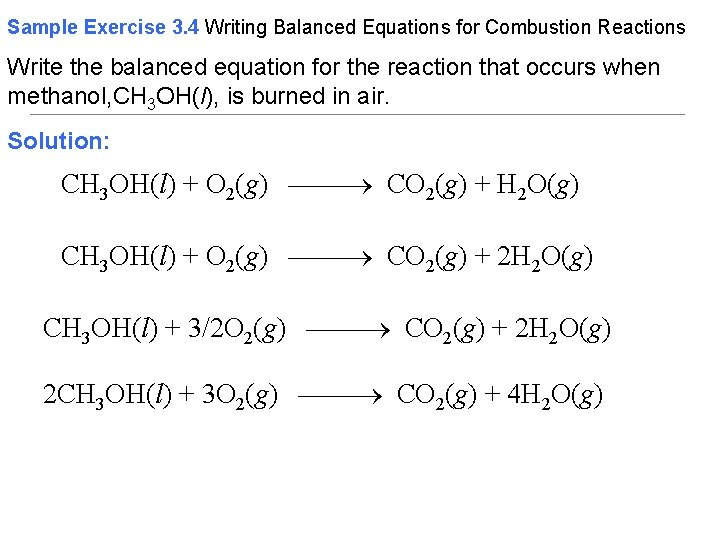Sample Exercise 3. 4 Writing Balanced Equations for Combustion Reactions Write the balanced equation for the reaction that occurs when methanol, CH 3 OH(l), is burned in air. Solution: CH 3 OH(l) + O 2(g) CO 2(g) + H 2 O(g) CH 3 OH(l) + O 2(g) CO 2(g) + 2 H 2 O(g) CH 3 OH(l) + 3/2 O 2(g) CO 2(g) + 2 H 2 O(g) 2 CH 3 OH(l) + 3 O 2(g) CO 2(g) + 4 H 2 O(g)Formula Weights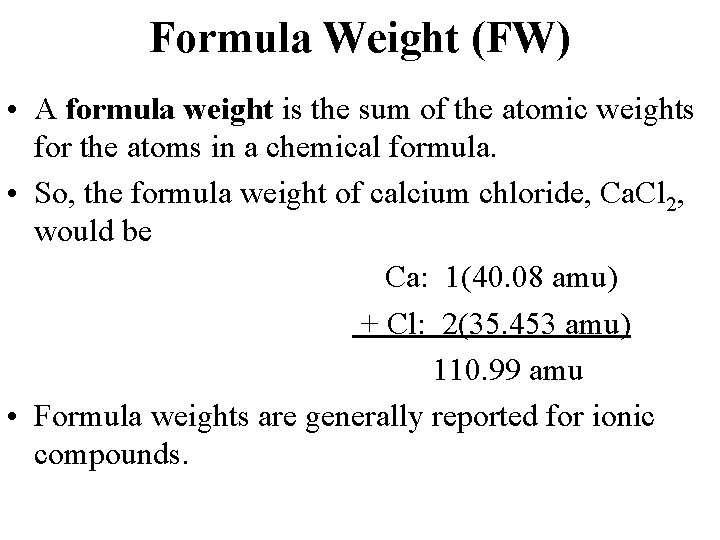Formula Weight (FW) • A formula weight is the sum of the atomic weights for the atoms in a chemical formula. • So, the formula weight of calcium chloride, Ca. Cl 2, would be Ca: 1(40. 08 amu) + Cl: 2(35. 453 amu) 110. 99 amu • Formula weights are generally reported for ionic compounds.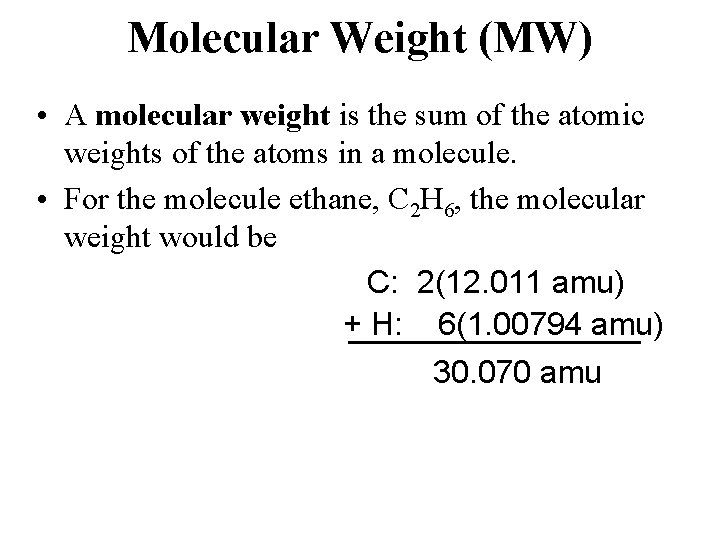Molecular Weight (MW) • A molecular weight is the sum of the atomic weights of the atoms in a molecule. • For the molecule ethane, C 2 H 6, the molecular weight would be C: 2(12. 011 amu) + H: 6(1. 00794 amu) 30. 070 amu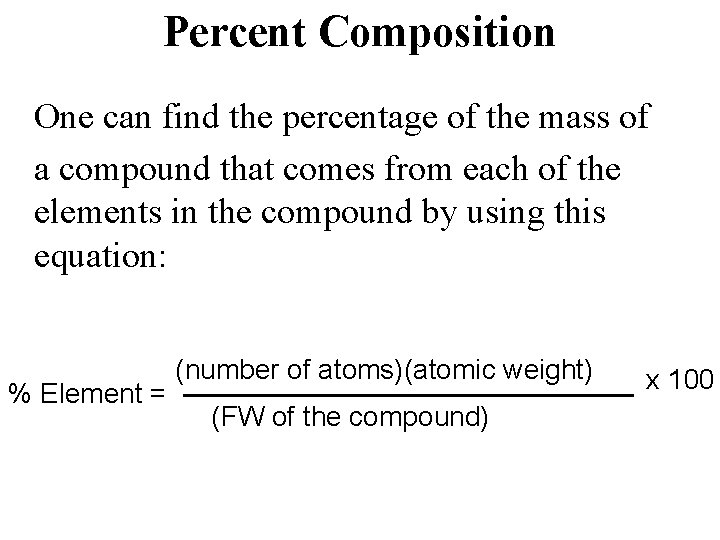Percent Composition One can find the percentage of the mass of a compound that comes from each of the elements in the compound by using this equation: % Element = (number of atoms)(atomic weight) (FW of the compound) x 100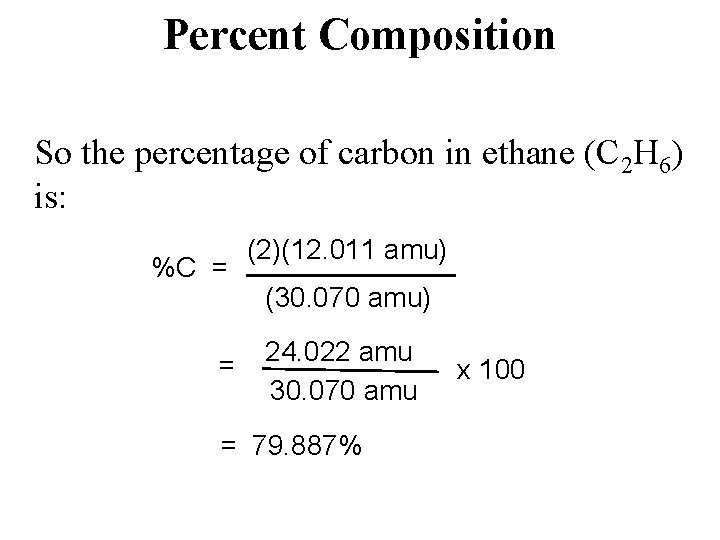Percent Composition So the percentage of carbon in ethane (C 2 H 6) is: %C = = (2)(12. 011 amu) (30. 070 amu) 24. 022 amu 30. 070 amu = 79. 887% x 100Moles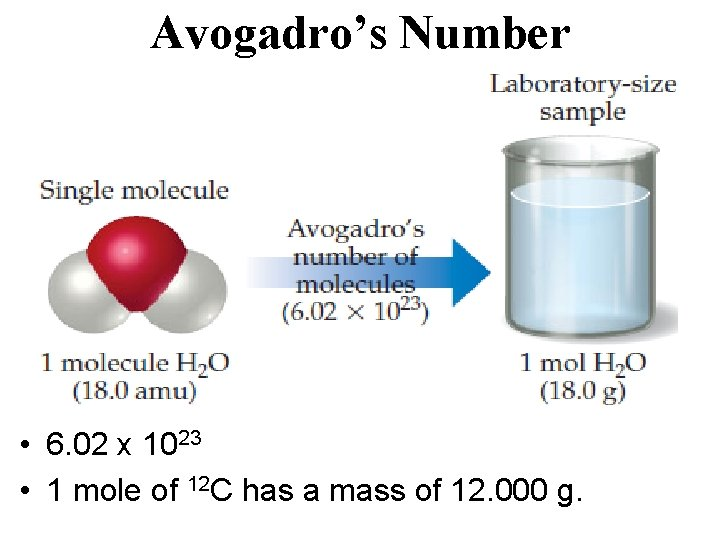Avogadro’s Number • 6. 02 x 1023 • 1 mole of 12 C has a mass of 12. 000 g.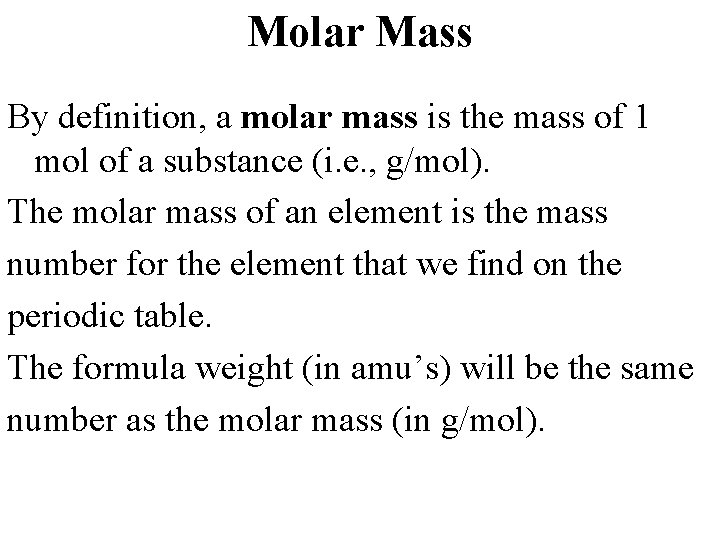Molar Mass By definition, a molar mass is the mass of 1 mol of a substance (i. e. , g/mol). The molar mass of an element is the mass number for the element that we find on the periodic table. The formula weight (in amu’s) will be the same number as the molar mass (in g/mol).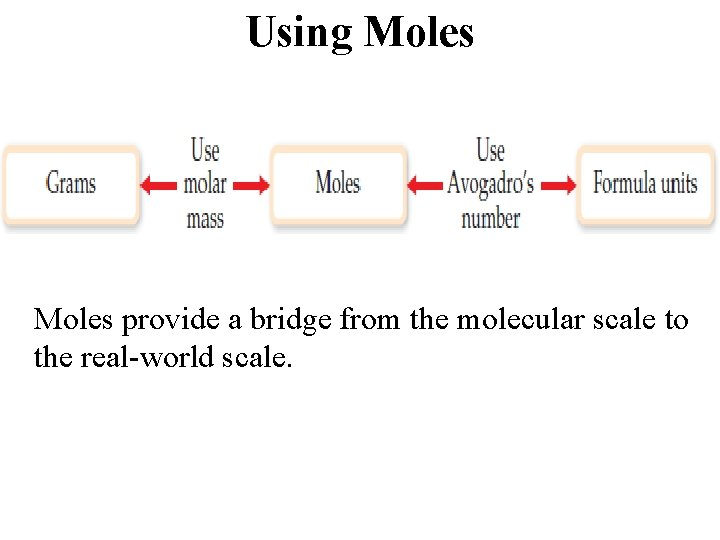Using Moles provide a bridge from the molecular scale to the real-world scale.Mole Relationships • One mole of atoms, ions, or molecules contains Avogadro’s number of those particles. • One mole of molecules or formula units contains Avogadro’s number times the number of atoms or ions of each element in the compound.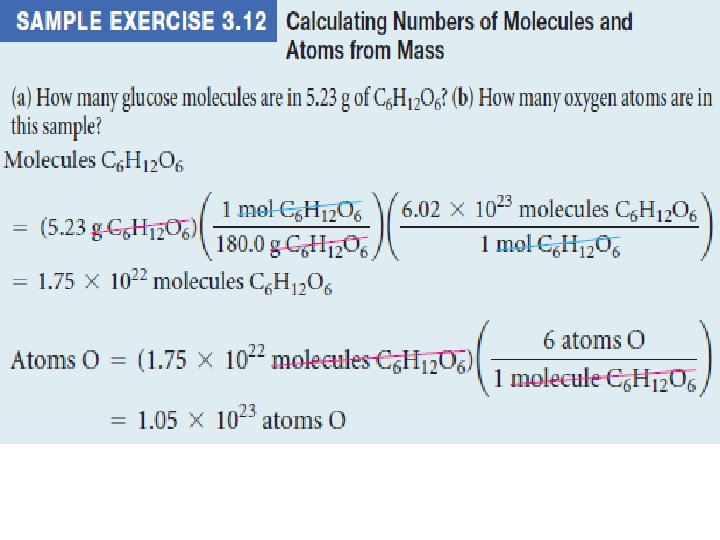Finding Empirical Formulas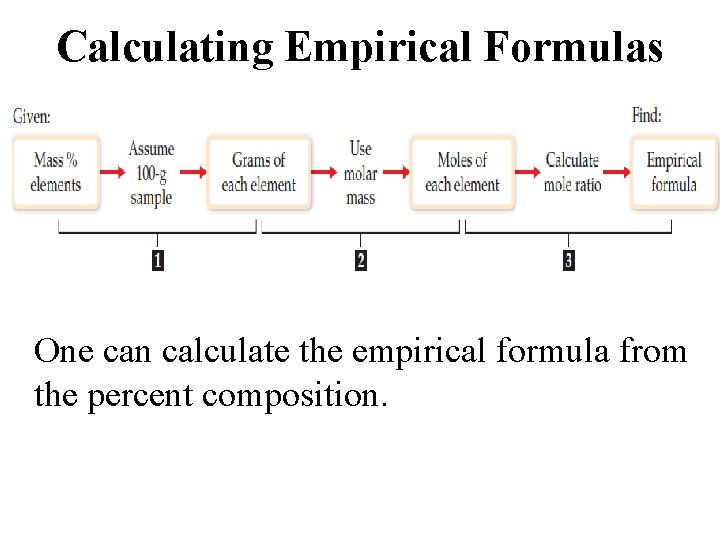Calculating Empirical Formulas One can calculate the empirical formula from the percent composition.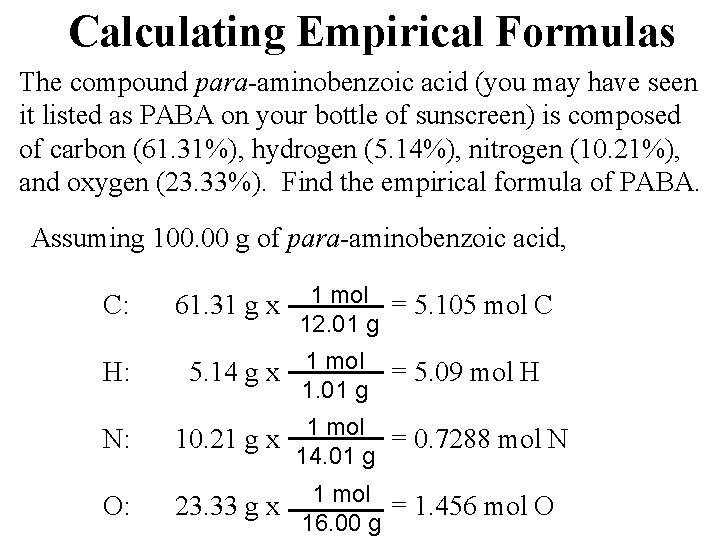Calculating Empirical Formulas The compound para-aminobenzoic acid (you may have seen it listed as PABA on your bottle of sunscreen) is composed of carbon (61. 31%), hydrogen (5. 14%), nitrogen (10. 21%), and oxygen (23. 33%). Find the empirical formula of PABA. Assuming 100. 00 g of para-aminobenzoic acid, C: 61. 31 g x 1 mol = 5. 105 mol C 12. 01 g H: 5. 14 g x 1 mol = 5. 09 mol H N: 1 mol 10. 21 g x = 0. 7288 mol N 14. 01 g O: 23. 33 g x 1. 01 g 1 mol = 1. 456 mol O 16. 00 g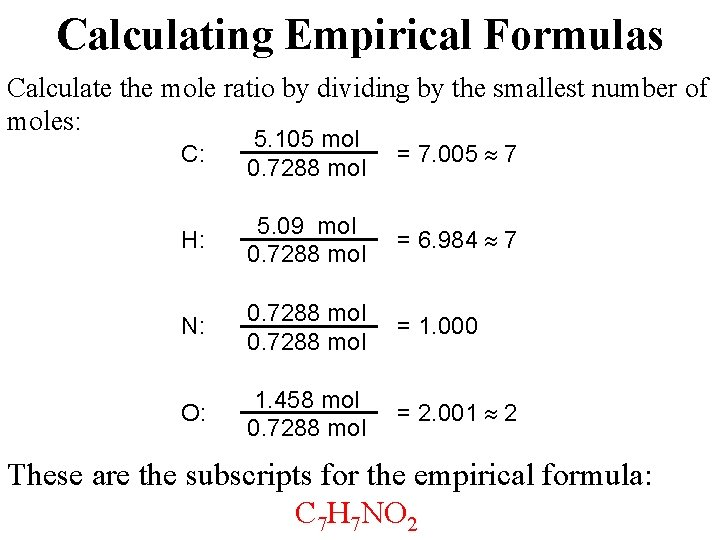Calculating Empirical Formulas Calculate the mole ratio by dividing by the smallest number of moles: C: 5. 105 mol 0. 7288 mol = 7. 005 7 H: 5. 09 mol 0. 7288 mol = 6. 984 7 N: 0. 7288 mol = 1. 000 O: 1. 458 mol 0. 7288 mol = 2. 001 2 These are the subscripts for the empirical formula: C 7 H 7 NO 2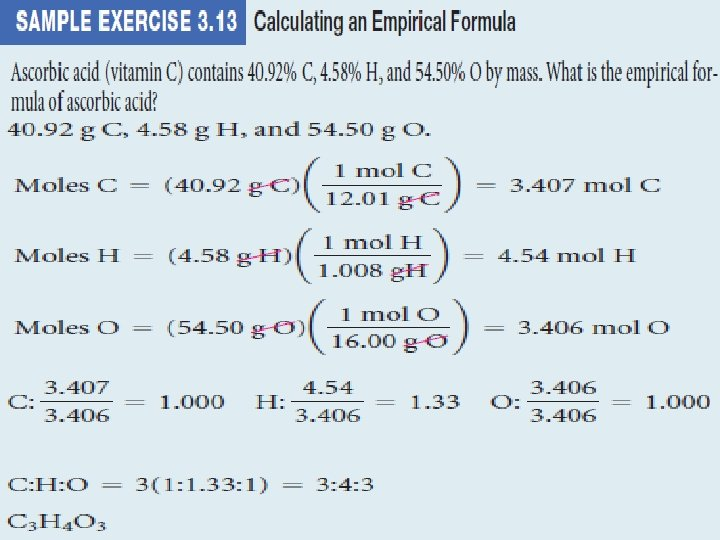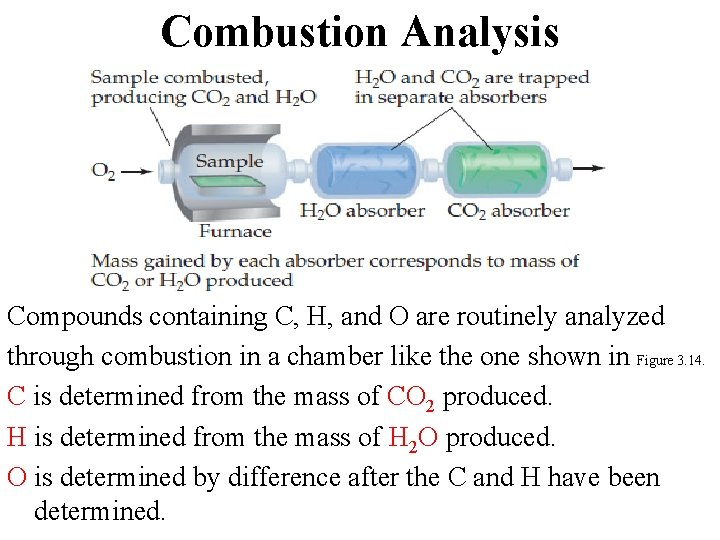Combustion Analysis Compounds containing C, H, and O are routinely analyzed through combustion in a chamber like the one shown in Figure 3. 14. C is determined from the mass of CO 2 produced. H is determined from the mass of H 2 O produced. O is determined by difference after the C and H have been determined.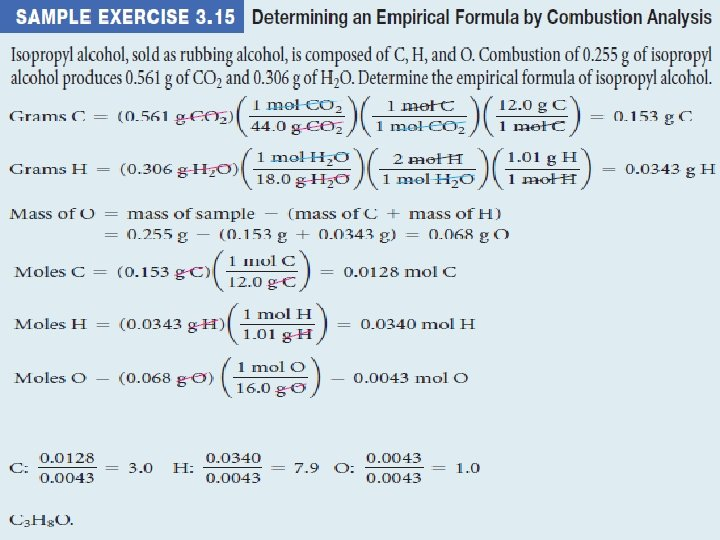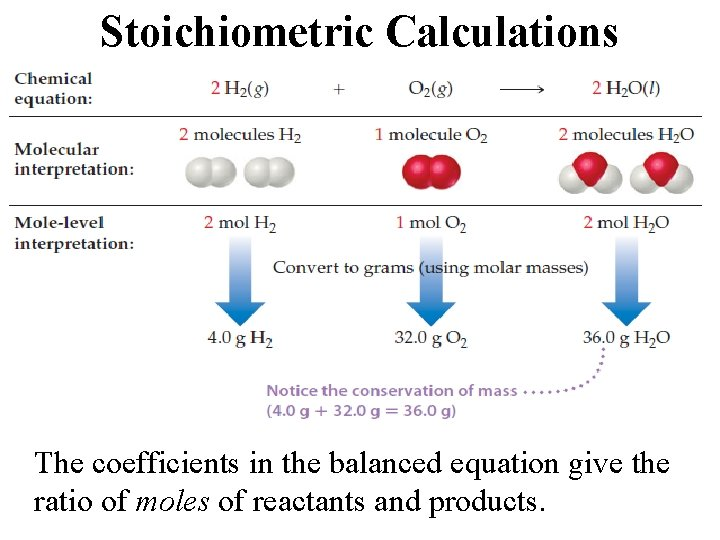Stoichiometric Calculations The coefficients in the balanced equation give the ratio of moles of reactants and products.Starting with the mass of Substance A, you can use the ratio of the coefficients of A and B to calculate the mass of Substance B formed (if it’s a product) or used (if it’s a reactant).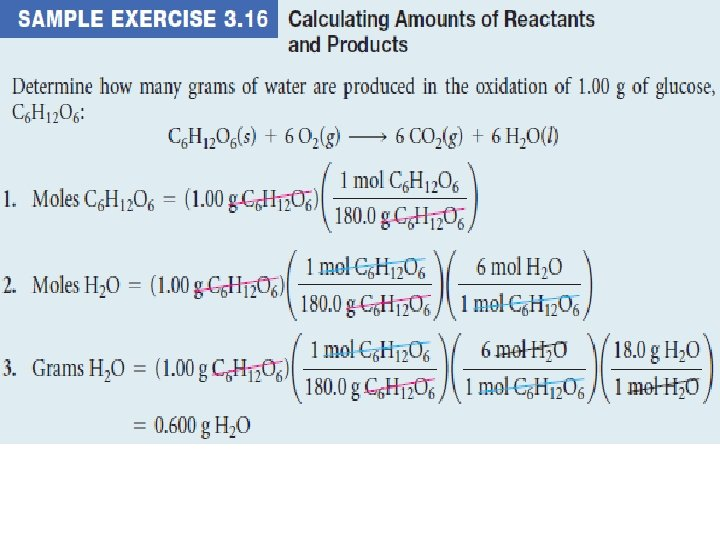C 6 H 12 O 6 + 6 O 2 6 CO 2 + 6 H 2 O Starting with 1. 00 g of C 6 H 12 O 6… we calculate the moles of C 6 H 12 O 6… use the coefficients to find the moles of H 2 O… and then turn the moles of water to grams.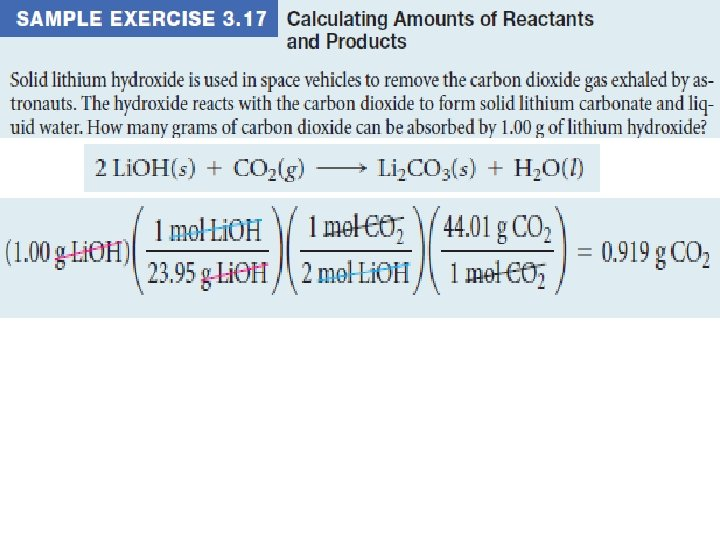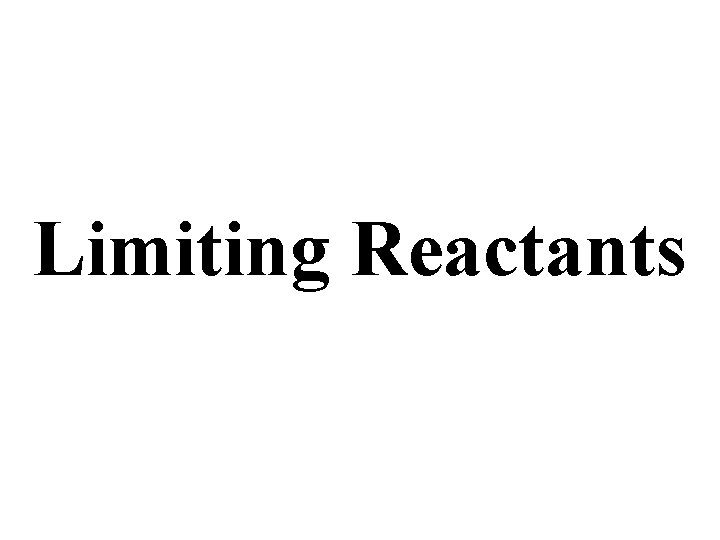Limiting ReactantsLimiting Reactants • The limiting reactant is the reactant present in the smallest stoichiometric amount. In other words, it’s the reactant you’ll run out of first (in this case, the H 2). The O 2 would be the excess reagent.Theoretical Yield • The theoretical yield is the maximum amount of product that can be made. – In other words, it’s the amount of product possible as calculated through the stoichiometry problem. • This is different from the actual yield, which is the amount one actually produces and measures.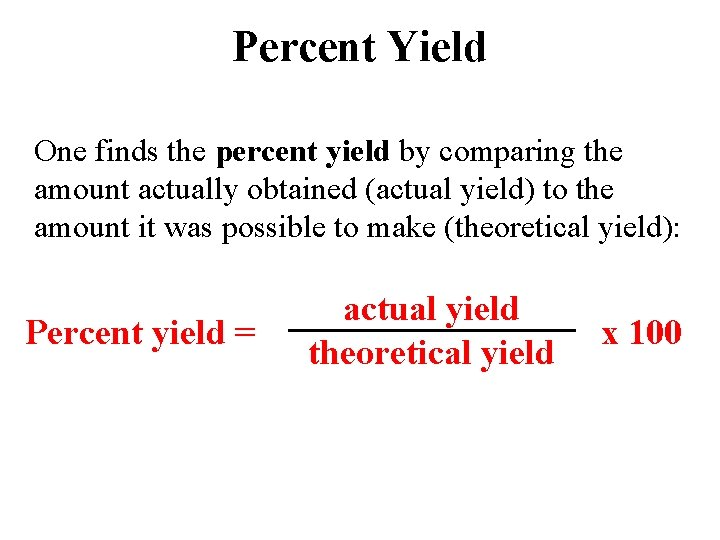Percent Yield One finds the percent yield by comparing the amount actually obtained (actual yield) to the amount it was possible to make (theoretical yield): Percent yield = actual yield theoretical yield x 100